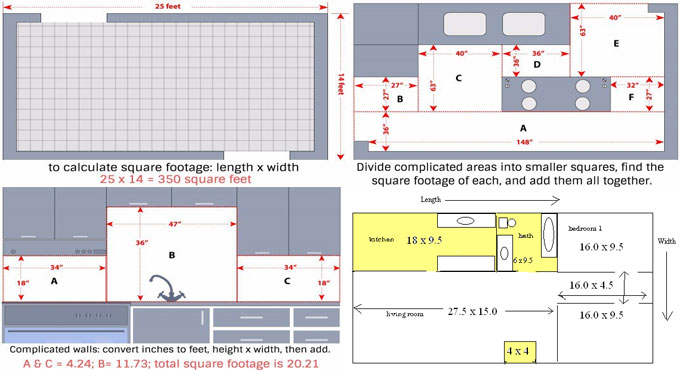# Some useful tips to work out the quantities of tiles for setting up tiles floorIf it is required to plan or set up your individual tile floor or just estimate the materials for budget purpose, you have to find out the quantities of tiles as per your need.

By using a measuring tape and some simple math, it is possible to calculate the quantities of tiles as well as total cost of the project.

For this purpose, you require the followings :-

? Paper
? Pen or pencil
? Measuring tape
? Calculator (optional)

The steps should be undertaken to determine the tiles quantities.

? Step 1

? Work out the length of one side of the room. For any wall project, calculate the length of the wall directing up and down.

? Step 2

? Work out the length of the other side of the room. For any wall project, calculate the length of the wall directing from left to right.

? Step 3

? Multiply the two measurements. Multiplying the length with the width will determine the square footage of the project area. As for instance, if the length of the room is 12 feet and width is 10 feet, then multiply 12 x 10. The square footage of this room will become 120 square feet.

? Step 4

? Translate the square footage to the amount of tile you need. Tile is generally found in boxes, and it is necessary to purchase the whole box. Divide the total square footage of the room with the total square footage of the tile in the box. Suppose, each box comprises of 10 square feet of tile. Therefore, for 120 square footage area, 10 will be 12 times in 120 and it is essential to purchase minimum 12 boxes.

? Step 5

? Calculate the average for your requirements. You should never purchase just the amount of tile you require, as you should require a little extra for cuts, waste, breaks, and mistakes. Multiply the square footage of the room by 10%, then include this amount to the total square of the room. This is the total amount of square footage that should have been purchase. As for example, 10 x 120 = 12, and 120 + 12 = 122. So, 122 square feet of tile will be required.

When the style or color is suspended and it is required to substitute a broken tile or two, you will require extras on hand for repairing purpose. For this purpose, you have to purchase extras. If extras are not purchased, then the entire floor should have been substituted.